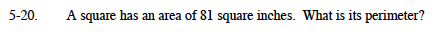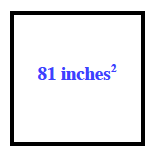### Home > MC1 > Chapter 5 > Lesson 5.1.2 > Problem5-20

5-20.Remember that perimeter is the distance around a shape. Also, a square has four sides which are equal in length.

Here is a sketch of a square with an area of 81 square inches. What number multiplied by itself equals 81? That number is the length of one side of the square.